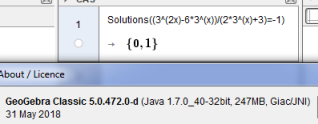# CAS cannot solve the equation

sigurdtorpnordby shared this problem 2 years ago
Solved

CAS gives no solution to this equation, although solving it by hand gives two solutions, x=0 and x=1. Also solving graphically, it's clear that there are two solutions.

(3^(2x)-6*3^(x))/(2*3^(x)+3)=-11

update version1

Aha! That's good.

I used the web-version and the latest updates of GeoGebra 5 and 6 from Mac App Store, where the problem still appear.